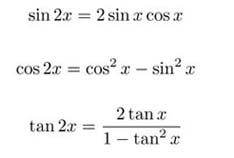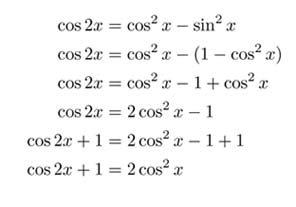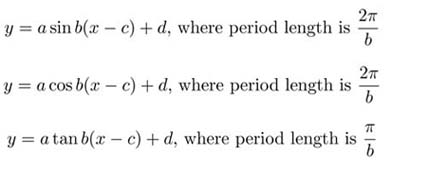SEARCH HOMEMath Central Quandaries & QueriesHodan, a student: Describe how you could use your knowledge of Double angle formulas to sketch the graph of each function. Include a sketch with your description. A) F(x)=sin x cos x B)F(x)=2 cos2x C) F(x)= tan(x) / ( 1-tan2x)Hi Hodan,

Here is a list of the double angle identities:You'll need to manipulate the fucntions a little bit to subsitute the double angle identity. For example part b)It will be much easier to graph f(x)=cos2x+1 because it is in stand graphing form now. When graphing trig functions, any coefficient infront of the x will effect the period of the function in the following waySo what is the period length of a double angle identity? How does this effect the appearance of the graph?

JaniceMath Central is supported by the University of Regina and The Pacific Institute for the Mathematical Sciences.